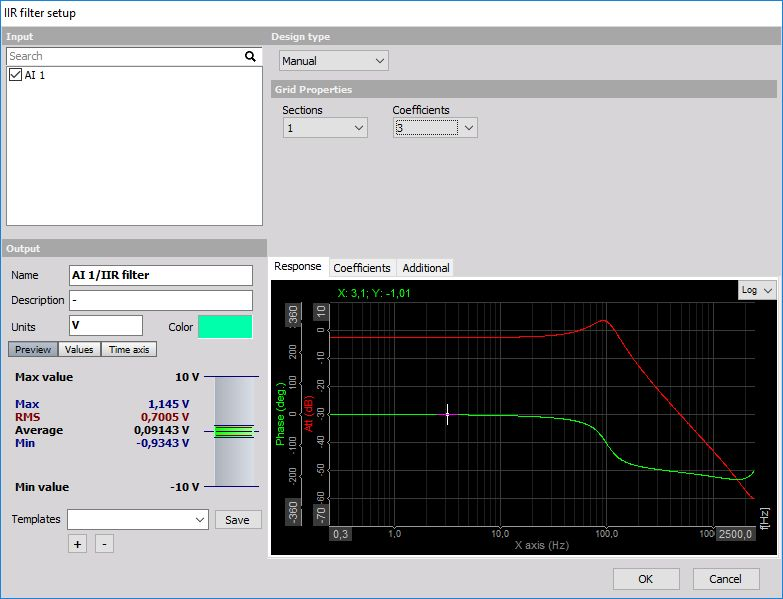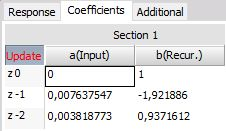# Custom filter - MANUAL

The Custom defined filter setup requires setting:

• number of Sections andCoefficients
• Scale

Individual filter coefficients value   It is possible to import custom filters - coefficients from Matlab, see -> Custom filter import from Matlab.

Usually, FIR filters consist from a single section. Multiple sections can be understood actually as several IIR filters combined into one (for example low pass and high pass). It also improves the stability of the filter since the coefficients are smaller. However, it is most common to use only one section for custom filters.

We define the number of coefficients per section which are the number of rows in the table. This basically defines the filter order.The last things to define are the filter coefficients. Enter a(input) and b(rekur.) values in the z-plane and press the Update button to change the filter settings. We can also copy/paste the coefficients from the clipboard by right-clicking and choosing ‘Copy to clipboard’ or ‘Paste from clipboard’ menu item.

The big question is now: how to define the coefficients. The answer to this question lies in the knowledge of filter design in s-plane and converting the filter to z-plane. We will try to shortly remember this procedure.

Usually, the filters are defined in the s-plane. Let’s take a simple example of the general formula for second order filter:

1. $$H(s) = \frac{g_0 + g_1 s + g_2 s^2}{h_0 + h_1 s + h_2 s^2}$$

To get the filter coefficients in the z-plane (time domain coefficients) we need to use the bilinear transformation:

1. $$s = 2 f_s \frac{1 + z^{-1}}{1 - z^{-1}}$$

where $f_s$ is the sampling frequency. The upper equation reveals the important fact of filters defined in the z plane - they work only for one sample rate. Therefore if we need the filters at different sampling rates, the coefficients need to be recalculated.

If we substitute the $s$ in equation (1) with the formula in equation (2), we get for the upper part:

1. $$g (1+z^{-1})^2 + 2 g_1 f_s (1-z^{-1}) (1+z^{-1}) + 4 g_2 {f_s}^2 (1-z^{-1})^2 = \\ (g_0 + 2 g_1 f_s + 4 g_2 {f_s}^2) + (2 g_0 - 8 g_2 {f_s}^2) z^{-1} + (g_0 - 2 g_1 f_s + 4 g_2 {f_s}^2) z^{-2}$$

The first third of the equation is valid for $z^0$ coefficient, second for $z^{-1}$ and the third one for $z^{-2}$ in the table above. The upper part of the equation (with $g$ coefficients) is valid for input part while the lower part with $h$ coefficients is valid for recursive part of the equation.

If we need higher order filter, we need to make equation similar to (3) with the larger number of the coefficients. The result will have also $z^{-3}$ factor.

Let’s now make a simple example for second order Butterworth filter. It has the following prototype in the s-plane:

1. $$H(s) = 1/\left( 1+ \sqrt{2} \frac{s}{\omega_c} + \left(\frac{s}{\omega_c}\right)^2 \right)$$

where the $ω_c$ is the cutoff frequency in rd/s. We have to adapt the cutoff frequency to the sample rate with pre warping:

1. $$\omega_c = 2 f_s \cdot \tan \left( \pi \frac{f_c}{f_s} \right)$$

If we write out the factors for this filter:

1. $$g_0 = 1; \quad g_1=0; \quad g_2 = 0; \\ h_0=1;\quad h_1= \frac{\sqrt{2}}{\omega_c}; \quad h_2=\left( \frac{1}{\omega_c} \right)^2$$

Now let’s make the following filter:

• cutoff frequency - $f_c = 100 \text{ Hz}$
• sampling rate - $f_s = 1000 \text{ Hz}$

First we do the pre warping:

$$f_{cp} = 2 \cdot 1000 \cdot \tan \left( \pi \frac{100}{1000} \right) = 649{,}8 \ \frac{\rm{rad}}{\rm{s}}$$

And now we need to calculate the coefficients for direct and recursive part of the filter with substituting factors (6) in equation (3):

$a(z^{0}) = 1+0+0 = 1$ $b(z^0) = 1 + 2 \cdot \sqrt{2} \cdot \frac{1000}{649{,}8} + 4 \cdot \frac{10002}{649{,}82} = 14{,}825$
$a(z^{-1}) = 2 \cdot 1-0 = 2$ $b(z^{-1}) = 2 \cdot 1 - 8 \cdot \frac{10002}{649{,}82} = -16{,}944$
$a(z^{-2}) = 1-0+0 = 1$ $b(z^{-2}) = 1 - 2 \cdot \sqrt{2} \cdot \frac{1000}{649{,}8} + 4 \cdot \frac{10002}{649{,}82} = 6{,}12$

Finally, we set the number of coefficients to 3, number of sections to 1 and enter calculated 6 values in the table and press Update. All entered values are red color and button Update also flash until Update is pressed.Remember, this is valid only for a sampling rate of 1000 Hz. For others, we need to recalculate the fcp and coefficients.

To make second-order Butterworth filter is much easier with Dewesoft standard filters, but if we need a specific filter, it is necessary to design it ‘by hand’.

The next chapter describes how to import custom filters from Matlab Custom filter import from Matlab.